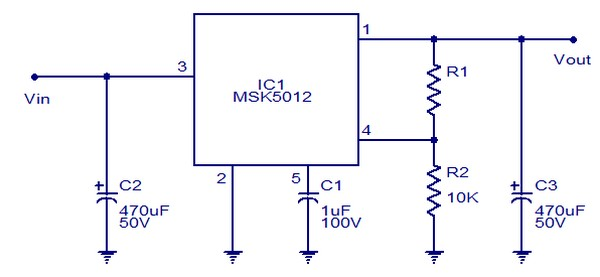## Monday, August 10, 2015

### Circuit Schematic 10A Adjustable voltage Regulator using MSK 5012 IC

Eltronicschool. - Here is one kind of voltage regulator circuit schematic with output more than 10 ampere. This is circuit schematic 10A Adjustable voltage Regulator using MSK 5012 IC. So, the based on this circuit schematic is MSK 5012 IC.

Circuit SchematicFigure 1. Circuit Schematic 10A Adjustable voltage Regulator using MSK 5012 IC (Circuitstoday)

Component Part
1. C1 = 1uF/100V
2. C2 = 470uF/50V
3. C3 = 470uF/50V
4. R1,R2 = 10K
5. IC1 = MSK5012

Description

Circuit schematic like in figure 1 above is circuit schematic 10A Adjustable voltage Regulator using MSK 5012 IC. The output voltage of this circuit is adjustable from 1.3v to 36v DC.Resistors R1 and R2 are used for programming the output voltage.For all applications, value of R2 is fixed to 10K. The relationship between R1,R2 and output voltage Vout is according to the equation R1=R2(Vout/1.235)-1. C1 is a filter capacitor which is also a part of the gate drive circuit of the internal series pass MOSFET. Around three times the input voltage will appear across this capacitor and so the its voltage rating must be selected accordingly.C2 is the input filter capacitor while C3 is the output filter capacitor.